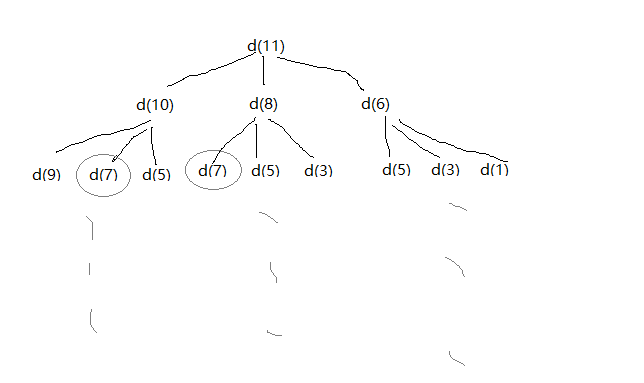# 问题：如果我们有面值为1元、3元和5元的硬币若干枚，如何用最少的硬币凑够11元？.md

### 问题

d(0) = 0 　　　　　　　　理所当然

d(1) = 1 　　　　　　　　要凑够1元，需要从面值小于等于1元的硬币中选择，目前只有面值为1元的硬币

此时d(1) = d(0) + 1

d(2) = d(2 - 1) + 1 = 2， 从面值小于等于2元的硬币中选择，符合要求的硬币面值为：1元。

此时d(2) = d(2-1) + 1

d(3) = d(3 - 3) + 1 = 1， 从面值小于等于3元的硬币中选择，符合要求的硬币面值为：1元，3元。

此时有有两种选择：是否选择含有面值3元的硬币

含有3元硬币：d(3) = d(3 - 3) + 1 = 1

不含3元硬币：d(3) = d(3 - 1) + 1 = d(2) + 1 = 3

自然是选择二者中较小值


### 算法思想

d(11) = min{d(10),d(8),d(6)} + 1

d(i) = min{d(i-v)} + 1,其中v为面值1，3，5；i为求值### 实现代码

/**
* https://www.cnblogs.com/z941030/p/4908115.html
* https://www.kancloud.cn/qiaodong/datastructure/134483#135_165
* DP
* @since 2019-10-25
*/
public class Solution_2019_11_9 {
// 如果我们有面值为1元、3元和5元的硬币若干枚，如何用最少的硬币凑够11元
public int select_coin(int[] coins, int total) {
// 状态转移方程
int[] minCoins = new int[total + 1];
minCoins = 0;

for (int i = 1; i <= total; i++) {
minCoins[i] = Integer.MAX_VALUE;
for (int j = 0; j < coins.length; j++) {
if (coins[j] <= i && minCoins[i - coins[j]] + 1 < minCoins[i]) {
minCoins[i] = minCoins[i - coins[j]] + 1;
}
}
}
System.out.println(Arrays.toString(minCoins));
return minCoins[total];
}

public static void main(String[] args) {
System.out.println(new Solution_2019_11_9().select_coin(new int[]{1, 3, 5}, 11));
}
}


©️2019 CSDN 皮肤主题: 技术黑板 设计师: CSDN官方博客